Related Articles
Delete rows and columns of NumPy ndarray
• Last Updated : 21 Apr, 2021

In this article, we will discuss how to delete the specified rows and columns in an n-dimensional array. We are going to delete the rows and columns using numpy.delete() method.

Syntax: numpy.delete(array_name, obj, axis=None)

Let’s discuss with the help of some examples:

Example 1:

Program to create a 2-dimensional array (3 rows and 4 columns) with NumPy and delete the specified row.

## Python3

 `# importing numpy module ` `import` `numpy as np ` ` `  `# create an array with integers ` `# with 3 rows and 4 columns ` `a ``=` `np.array([[``1``, ``2``, ``3``, ``4``], ` `              ``[``5``, ``6``, ``7``, ``8``],  ` `              ``[``9``, ``10``, ``11``, ``12``]]) ` `print``(a) ` ` `  `# delete 0 th row ` `data ``=` `np.delete(a, ``0``, ``0``) ` `print``(``"data  after 0 th row deleted :"``, data) ` ` `  `# delete 1 st row ` `data ``=` `np.delete(a, ``1``, ``0``) ` `print``(``"data  after 1 st  row deleted :"``, data) ` ` `  `# delete 2 nd row ` `data ``=` `np.delete(a, ``2``, ``0``) ` `print``(``"data  after 2 nd  row deleted :"``, data) `

Output: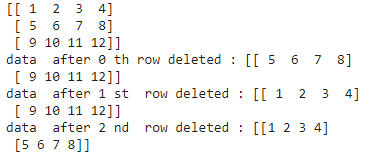Example 2:

Program to create a 2-dimensional array (6 rows and 2 columns) with NumPy and delete the specified columns.

## Python3

 `# importing numpy module ` `import` `numpy as np ` ` `  `# create an array with integers with ` `# 6 rows and 2 columns ` `a ``=` `np.array([[``1``, ``2``], [``5``, ``6``], [``9``, ``10``, ], ` `              ``[``78``, ``90``], [``4``, ``89``], [``56``, ``43``]]) ` `print``(a) ` ` `  `# delete 0 th column ` `data ``=` `np.delete(a, ``0``, ``1``) ` `print``(``"data  after 0 th  column  deleted :"``, data) ` ` `  `# delete 1 st column ` `data ``=` `np.delete(a, ``1``, ``1``) ` `print``(``"data  after 1 st  column  deleted :"``, data) `

Output: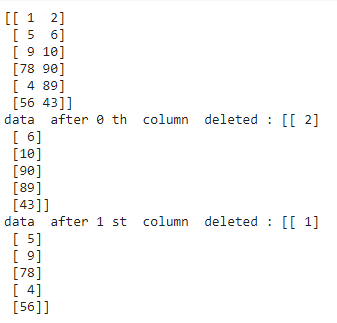Example 3:

Delete both 1 row and 1 column.

## Python3

 `# importing numpy module ` `import` `numpy as np ` ` `  `# create an array with integers ` `# with 3 rows and 3 columns ` `a ``=` `np.array([[``67``, ``65``, ``45``],  ` `              ``[``45``, ``67``, ``43``],  ` `              ``[``3``, ``4``, ``5``]]) ` `print``(``"Original\n"``, a) ` ` `  `# delete 1 st row ` `data ``=` `np.delete(a, ``0``, ``0``) ` `print``(``"data  after 1 st row   deleted :\n"``, data) ` ` `  `# delete 1 st column ` `data ``=` `np.delete(a, ``0``, ``1``) ` `print``(``"data  after 1 st  column  deleted :\n"``, data) `

Output: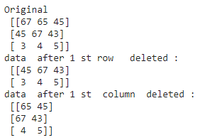Example 4:

We can delete n number of rows at a time by passing row numbers as a list in the obj argument.

Syntax: numpy.delete(array_name, [row1,row2,.row n], axis=None)

## Python3

 `# importing numpy module ` `import` `numpy as np ` ` `  `# create an array with integers  ` `# with 3 rows and 3 columns ` `a ``=` `np.array([[``67``, ``65``, ``45``],  ` `              ``[``45``, ``67``, ``43``],  ` `              ``[``3``, ``4``, ``5``]]) ` `print``(``"Original\n"``, a) ` ` `  `# delete 1 st row and 2 nd  ` `# row at a time ` `data ``=` `np.delete(a, [``0``, ``1``], ``0``) ` `print``(``"data  after 1 st  and 2 ns row deleted :\n"``, data) `

Output: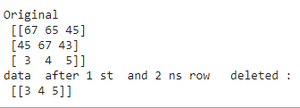Example 5:

We can delete n number of columns at a time by passing column numbers as a list in the obj argument.

Syntax: numpy.delete(array_name, [column number1,column number2,.column number n], axis=None)

## Python3

 `# importing numpy module ` `import` `numpy as np ` ` `  `# create an array with integers  ` `# with 3 rows and 3 columns ` `a ``=` `np.array([[``67``, ``65``, ``45``], ` `              ``[``45``, ``67``, ``43``],  ` `              ``[``3``, ``4``, ``5``]]) ` `print``(``"Original\n"``, a) ` ` `  ` `  `# delete 1 st column and 3 rd  ` `# column at a time ` `data ``=` `np.delete(a, [``0``, ``2``], ``1``) ` `print``(``"data  after 1 st and 3 rd column  deleted :\n"``, data) `

Output: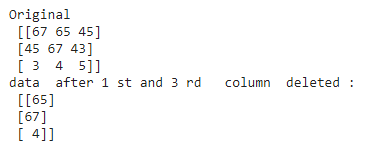Attention geek! Strengthen your foundations with the Python Programming Foundation Course and learn the basics.

To begin with, your interview preparations Enhance your Data Structures concepts with the Python DS Course. And to begin with your Machine Learning Journey, join the Machine Learning – Basic Level Course

My Personal Notes arrow_drop_up
Recommended Articles
Page :